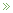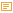• Maximum Likelihood for Incomplete Data

Posted by Konstantin 13.11.2008

It is good to attend lectures from time to time, even if these are on the topics that you "already know". Because, once in a while, you get to find out something new about things that are so "simple" and "familiar", that you thought there was nothing new you could possibly discover about them. So, today I've had an accidental revelation regarding a simple yet amusing property of the maximum likelihood estimation method. I think I should have been told that years ago, right on the first statistics course.

Maximum Likelihood

Maximum likelihood estimation is one of the simplest and most popular methods for fitting mathematical models to given data. It is most easily illustrated by an example. Suppose you are given a list of numbers, which, you believe, are all instances of a normally distributed random variable with mean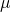and variance. The problem is that the value ofis unknown to you and you would like to estimate it from the data. How do you do it? The maximum likelihood method suggests you to search for a value of, for which the probability (or probability density) of obtaining exactly this set of numbers would be maximal. That is: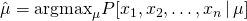In most cases this turns out to be quite a reasonable suggestion, which leads to good estimates (although, there are some rare exceptions). Here, for example, it turns out that to estimate the mean you should simply compute the average of the given numbers:This is more-or-less all what a typical textbook has to say about maximal likelihood, but there's more to it.

Counting Lightbulbs

Consider the following example. Suppose you are willing to measure the average lifetime of a common lightbulb (that is, the amount of time it will glow until burning out). For that you buy 1000 lightbulbs in a general store, switch all of them on and wait for a year, keeping track of the timepoints at which the  individual lightbulbs burn out. Suppose the year has passed and you managed to record the lifetime of just 50 "dead" lightbulbs (the remaining 950 keep on glowing even after the whole year of uninterrupted work). What do you do now? Do you wait for other lightbulbs to burn out? But this can take ages! Do you just discard the 950 lightbulbs and only consider the 50 for which you have "available data"? This is also not good, because, clearly, the knowledge that 95% of lightbulbs live longer than a year is important. Indeed, from this fact alone you could infer that the average lifetime must be between 18 and 21 years with 95% confidence (an interested reader is invited to verify this claim). So what do you do?

Assume that the lifetime of a lightbulb can be modeled using exponential distribution with mean(this is a natural assumption because exponential distribution is well-suited for describing the lifetime of electric equipment). Then the probability (probability density, in fact) that lightbulb i burns out after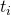years can be expressed as: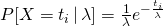.

Naturally, the likelihood of lightbulbs 1,2,3,...,50 burning out after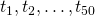years correspondingly, is just the product of the corresponding expressions: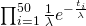.

Now what about the lightbulbs that are still alive? The likelihood that a lightbulb will glow longer than a year can be easily expressed as: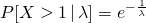.

Therefore, the total likelihood of having 50 lightbulbs burn out at time momentsand 950 lightbulbs keep working longer than a year is the following product: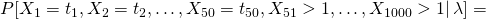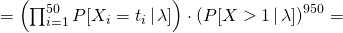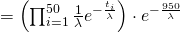.

Finding the estimate ofthat maximizes this expression is now a simple technical detail of minor importance. What is important is the ease with which we managed to operate the "uncertain measurements" for the 950 lightbulbs.

Final Remarks

Note that the idea can be extended to nearly arbitrary kinds of uncertainty. For example, if, for some lightbulb we knew that its lifetime was a number between a and b, we would include this piece of knowledge by using the term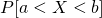in the likelihood function. This inherent flexibility of the maximum likelihood method looks like a feature quite useful for the analysis of noisy data.

Surprisingly, however, it does not seem to be applied much in practice. The reason for that could lie in the fact that many nontrivial constraints on the data will make the likelihood function highly nonconvex and hard to optimize. Consider, for example, the probability densityfor a normally distributed variable X. Depending on the choice of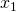,, and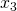, the likelihood function will have either three different peaks at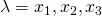, two peaks or just one. Thus, finding an exact optimum is much harder than in the typical textbook case. But on the other hand, there are various cases where the likelihood function is nicely convex (consider the interval constraint mentioned above) and besides there are numerous less efficient algorithms around anyway. So maybe the trick is not used much, simply because it's not in the textbook?

Posted by Konstantin @ 9:06 pm

• 1 Comment

1. -Jaak on 01.12.2008 at 14:31 (Reply)

In a random episode of show on Discovery channel (people were constructing things) they paid a visit to a fire station, that had a lightbulb that had been glowing constantly for the last 105+ years! Now, that's some technology!

Please note: Comment moderation is enabled and may delay your comment. There is no need to resubmit your comment.

Calendar

November 2008
M T W T F S S
« Oct   Dec »
12
3456789
10111213141516
17181920212223
24252627282930